# Rota's unimodal conjecture

 Importance: High ✭✭✭
 Author(s): Rota, Gian-Carlo
 Subject: Combinatorics » Matroid Theory
 Keywords: flat log-concave matroid
 Posted by: mdevos on: June 8th, 2007

Let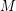be a matroid of rank, and for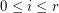let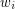be the number of closed sets of rank.

Conjectureis unimodal.
Conjectureis log-concave.

A sequence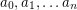is log-concave if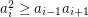for all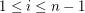.

The first of these conjectures is due to Rota [R], the second is folklore as far as I (M. DeVos) know. The special case of proving the second conjecture for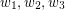amounts to showing thatand has been called the points-lines-planes conjecture. Seymour [S] proved this conjecture in the special case where every line contains at most four points, but it is still open in general.

## Bibliography

*[R] Rota, Gian-Carlo, Combinatorial theory, old and new. Actes du Congrès International des Mathématiciens (Nice, 1970), Tome 3, pp. 229--233. Gauthier-Villars, Paris, 1971. MathSciNet

[S] Seymour, P. D. On the points-lines-planes conjecture, J. Combin. Theory Ser. B 33 (1982), no. 1, 17--26. MathSciNet

* indicates original appearance(s) of problem.